# Number Base Conversion in JavaScript

## Converting from one base to another in JavaScript is easier than you thoughtPhoto by Sigmund on Unsplash

Did you know that converting from one base to another in JavaScript is easier than you thought?

All you need to master are just two functions. Are you wondering what those functions are? They are `parseInt()` and `toString()`.

• What is `parseInt()`?

• What is `toString()`?

• How to convert from other bases to base 10 (Decimal)

• How to convert from base 10 (Decimal) to other bases

• How to convert from a base other than base 10 (Decimal) to another base.

### What is parseInt()?

`ParseInt()` is a JavaScript built-in function that takes in `string` and `radix` as an argument and returns an equivalent decimal of the string in the specified radix.

``````parseInt(string, radix)
``````

The string parameter is required while the radix is optional. But to use it for base conversion the two parameters are required.

Let's dive into an example.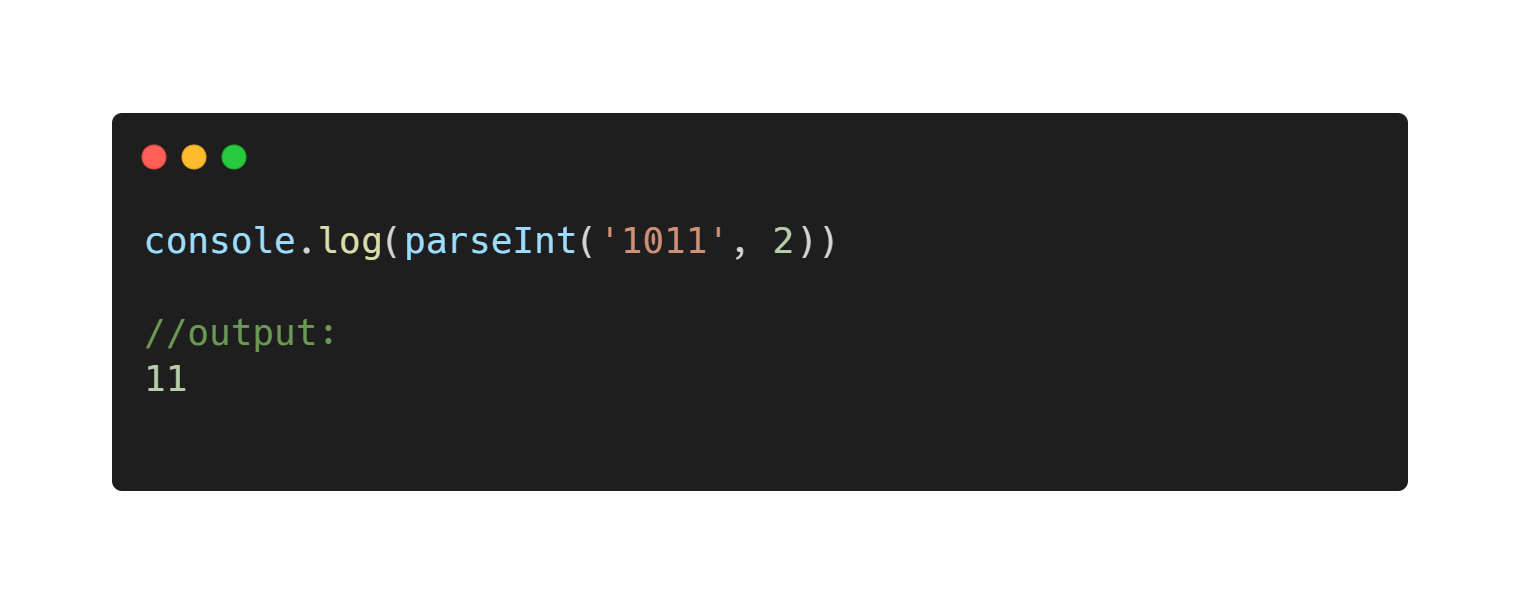Looking at this example, the string parameter takes in 1011 which is a string and the radix (base) of the number is 2.

The code converted 1011 base 2 to base 10.

### What is toString()?

`toString()` is a JavaScript method that returns the string conversion of the data it is used on.

For number base conversion, `toString()` converts a decimal to the specified base(radix).

Let's dive into an example.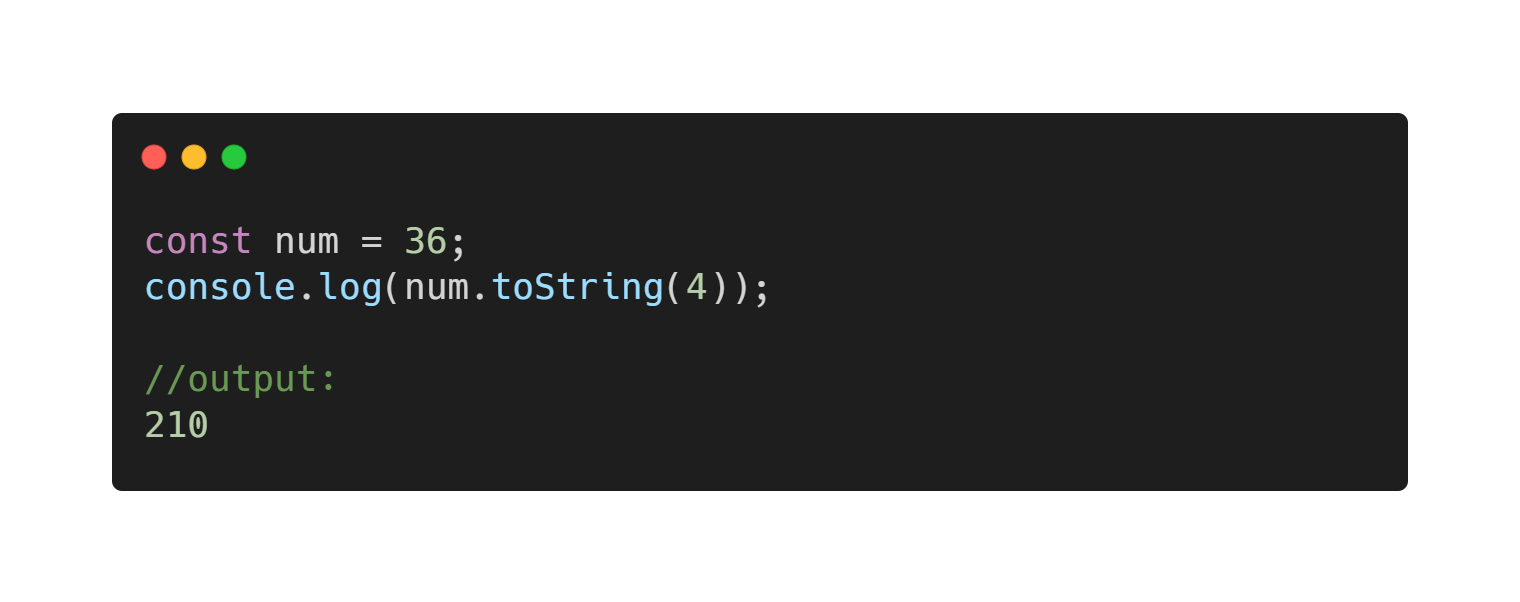The above code snippet shows `toString()` is used on `num=36` and takes in `4` as its parameter which is the radix.

The code converted 36 in base 10 to 210 in base 4.

Now, this is important.

### How to convert from other bases to base 10(Decimal)

Remember, `parseInt()` is used for converting from other bases to base 10 (decimal).

Let's dive into an example.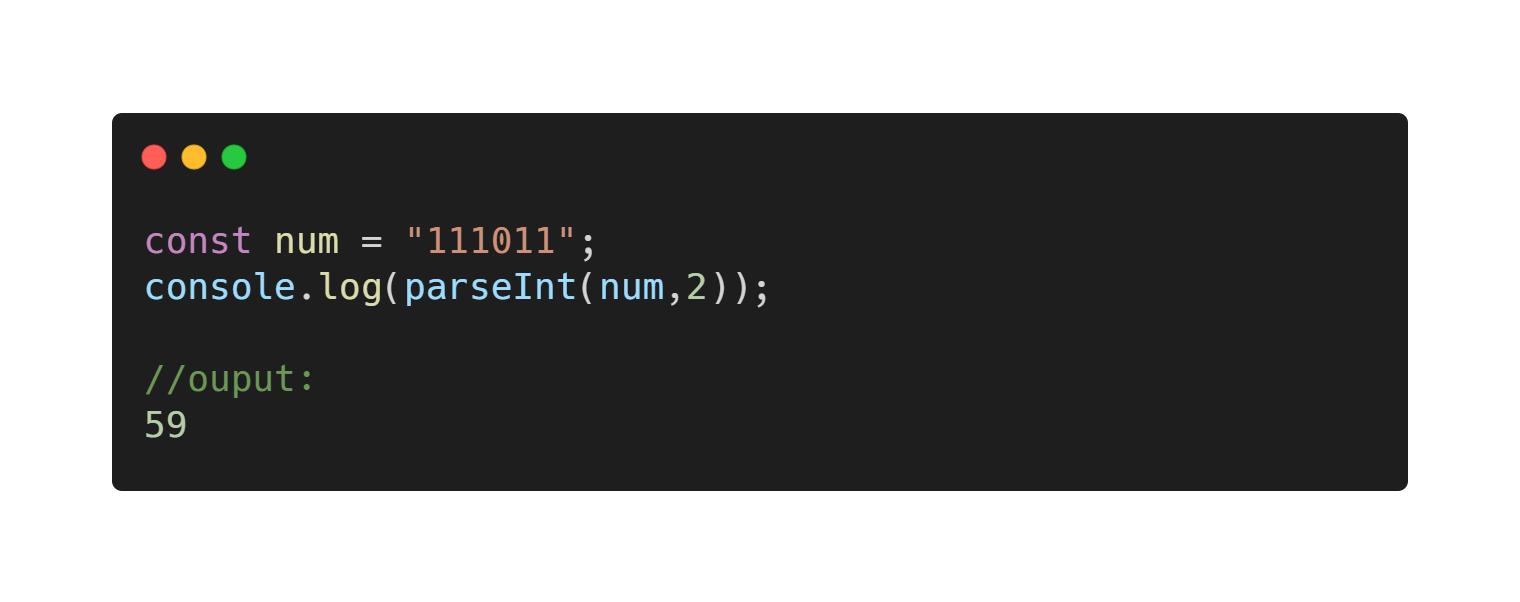In the code snippet above, `num` is passed in as a string with the `radix` of 2 and 59 is produced as the equivalence in decimal.

Are you confused? Let's take a dive into another case.`parseInt()` takes in 436 which is a string as its first parameter and 8 which is the radix as the second parameter then converted it to 286 in base 10(decimal).

Do you get it?

Now, Let's move to the next one.

### How to convert from base 10(Decimal) to other bases

To convert from base 10 i.e decimal to other bases all you need is the `toString()` method.

Let's dive into an example.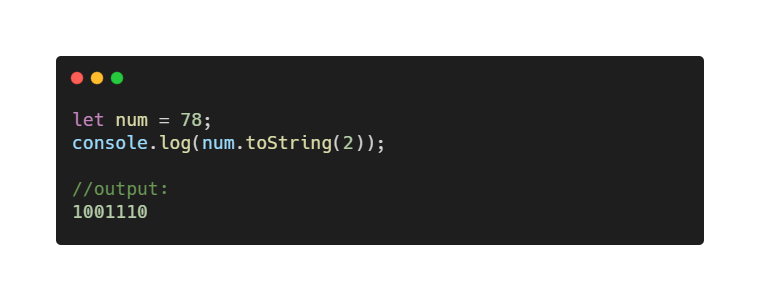The code snippet above shows `num` has the value of 78 which is in base 10. The 78 in the `num` is then converted to base 2 which is the specified `radix` in the `toString()` method.

Is it confusing? Let's take a dive into another case.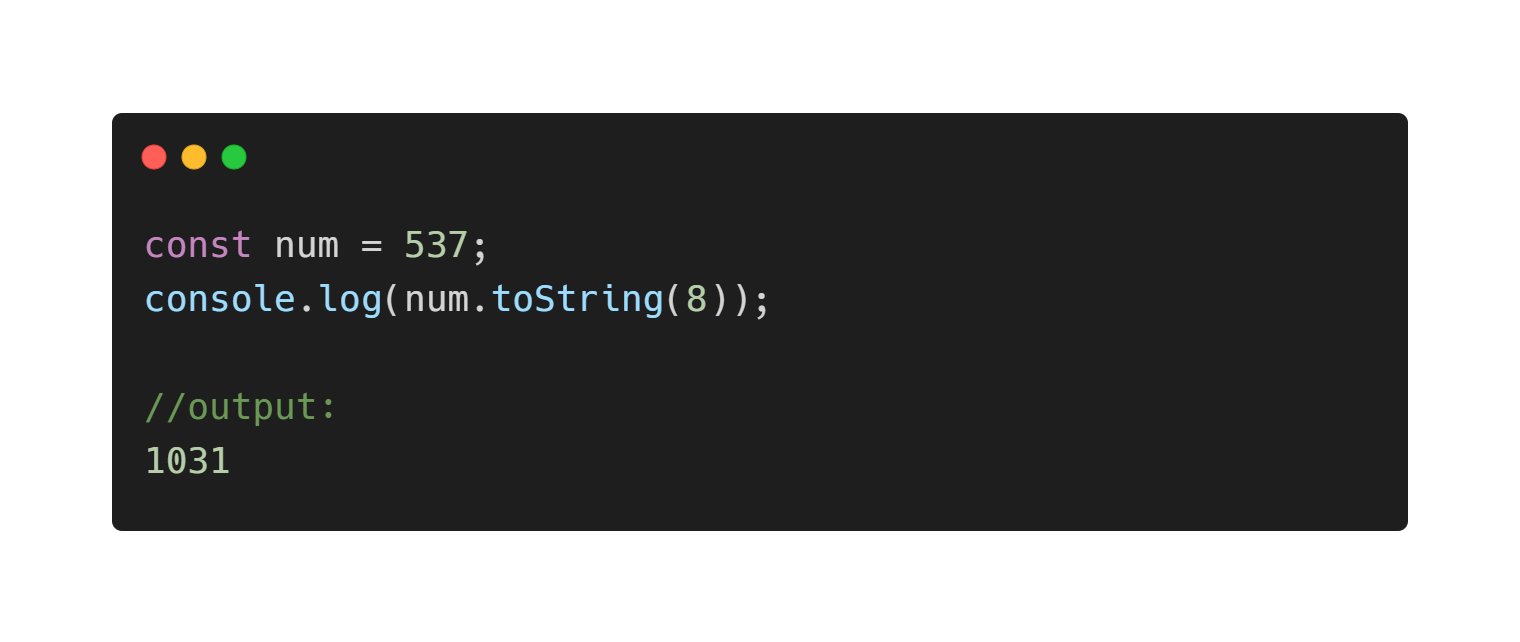In the code snippet above, the `toString()` method is used on `num` which is a decimal (base 10) number and it takes in a radix of 8 as its parameter.

The code is simply converting 537 in decimal to 1031 in octal (base 8).

### How to convert from a base other than base 10(Decimal) to another

All we have been doing is converting from base 10 to other bases and vice versa.

Is it possible to convert from base 3 to base 8? Or from base 7 to base 2?

Yeah! It is possible.

To convert from a base(other than 10) to another, all you have to do is convert the number to base 10 using `parseInt()`, then convert the base 10 number to the required base using `toString()`.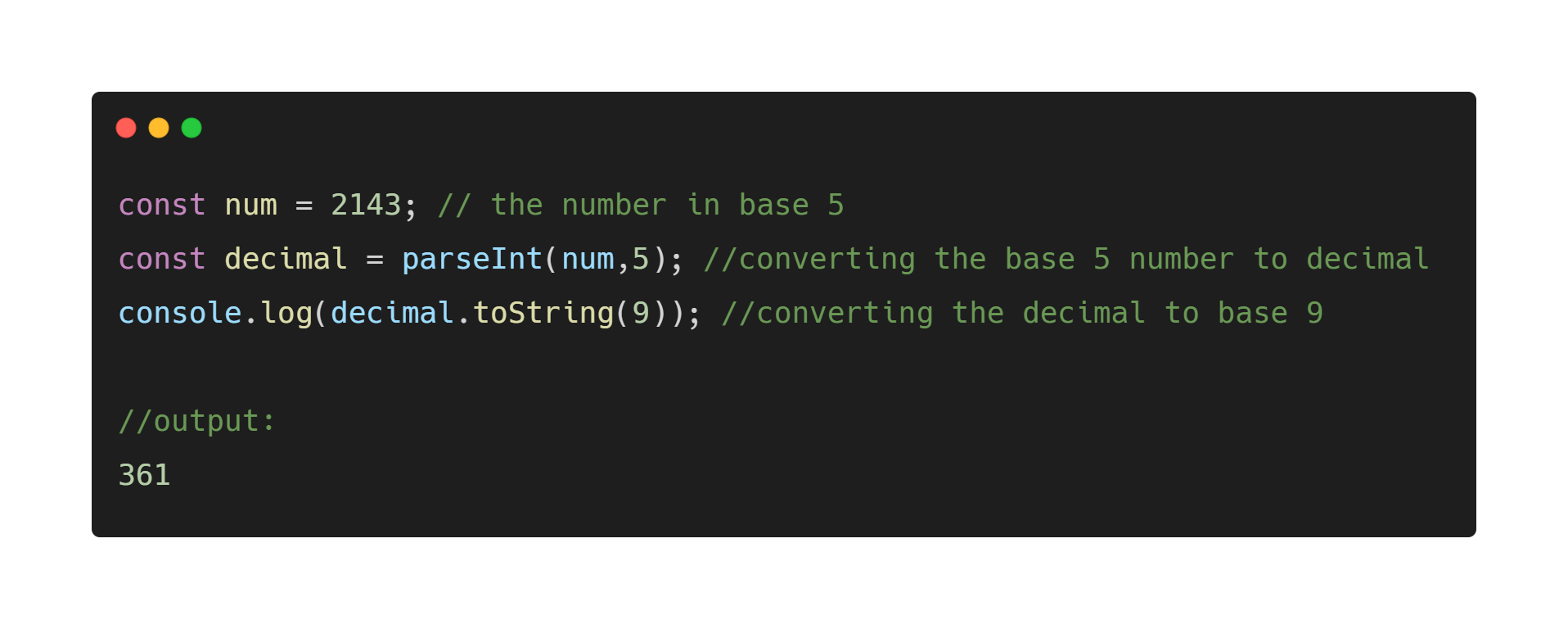The code snippet above shows how to convert from base 5 to base 9. The base 5 number is first converted to base 10 using `parseInt()`, then later converted the base 10 to base 9 using `toString()`.

Yeah! You finally made it to the end. Thanks for reading. I hope you found this useful.